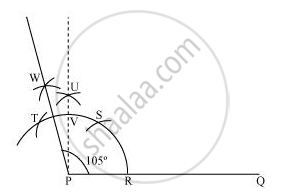# Construct the 105° angles and verify by measuring it by a protractor. - Mathematics

Construct the 105° angles and verify by measuring it by a protractor.

#### Solution

The below given steps will be followed to construct an angle of 105°.

(1) Take the given ray PQ. Draw an arc of some radius taking point P as its centre, which intersects PQ at R.

(2) Taking R as centre and with the same radius as before, draw an arc intersecting the previously drawn arc at S.

(3) Taking S as centre and with the same radius as before, draw an arc intersecting the arc at T (see figure).

(4) Taking S and T as centre, draw an arc of same radius to intersect each other at U.

(5) Join PU. Let it intersect the arc at V. Taking T and V as centre, draw arcs with radius more than 1/2TV. Let these arcs intersect each other at W. Join PW which is the required ray making 105° with the given ray PQ.The angle so formed can be measured with the help of a protractor. It comes to be 105º.

Concept: Basic Constructions
Is there an error in this question or solution?

#### APPEARS IN

NCERT Class 9 Maths
Chapter 11 Constructions
Exercise 11.1 | Q 4.2 | Page 191